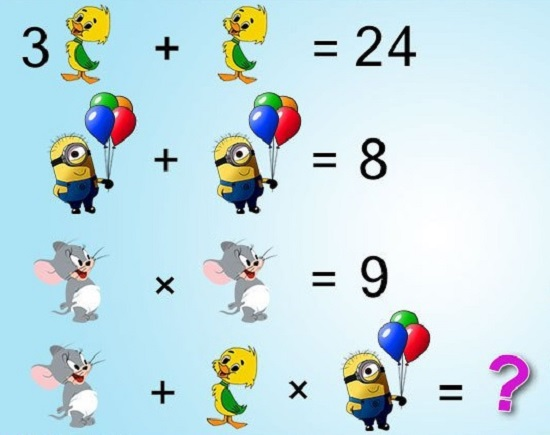## Whatsapp Math Number Puzzles with Answers & Step by Step Procedure:

Solve Whatsapp Math Quiz (Animals)

### Question 1: People have been asking this question on Whatsapp: Find answer of the following Animals (Math) Puzzle:

Bird + Rabbit = 5 kg
Pig – Bird = 99 kg
Rabbit + Rabbit + Rabbit = 12 kg
Bird + Pig + Rabbit = ?

#### Equation 3:

Rabbit + Rabbit + Rabbit = 12 kg
3 Rabbit = 12 kg
Rabbit = 4 kg

#### Equation 1:

Bird + Rabbit = 5 kg
Bird + 4 kg = 5 kg
Bird = 1 kg

#### Equation 2:

Pig – Bird = 99 kg
Pig – 1 kg = 99 kg
Pig = 100 kg

#### Equation 4:

Bird + Pig + Rabbit = ?
1 kg + 100 kg + 4 kg = ?

### Question 2: Another question I saw on Whatsapp & it is about solving cartoon (Math) Quiz:Solve Cartoon Math Quiz

#### Equation 1:

3 Duckling + Duckling = 24
4 Duckling = 24
Duckling = 6

#### Equation 2:

Character (with 4 balloons) + Character (with 4 balloons) = 8
2 Character (with 4 balloons) = 8
Character (with 4 balloons) = 4

#### Equation 3:

Mouse x Mouse = 9
Mouse^2 = 9
Mouse = 3

#### Equation 4:

Mouse + Duckling x Character (with 3 balloons) = ?

Note: There are 3 balloons not 4.

3 + 6 x 3 = ?